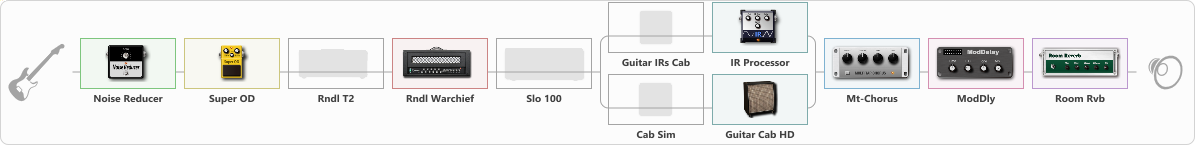# Distortion for phones

Discussion in 'ToneLib-GFX presets' started by Дворядкин Павел, Mar 16, 2023.

1. ### Дворядкин ПавелNew Member

Distortion for phones

Preset name: Distortion for phones

Effects chain:Effect: "Noise Reducer" (Dynamics / Filter), active - "yes"
{
"Sens" = 100
"Mode" = Soft
}

Effect: "Super OD" (Overdrive / Distortion), active - "yes"
{
"Drive" = 0
"Tone" = 80
"Level" = 97
}

Effect: "Rndl T2" (Amp simulators), active - "no"
{
"Gain" = 70
"Bass" = 100
"Middle" = 50
"Treble" = 50
"Presence" = 100
"Master" = 50
"Level (dB)" = 8
}

Effect: "Rndl Warchief" (Amp simulators), active - "yes"
{
"Gain" = 40
"Bass" = 100
"Middle" = 50
"Treble" = 50
"Presence" = 50
"Master" = 50
"Level (dB)" = 7
}

Effect: "Slo 100" (Amp simulators), active - "no"
{
"Gain" = 75
"Bass" = 40
"Middle" = 57
"Treble" = 35
"Presence" = 10
"Master" = 69
"Level (dB)" = 0
}

Effect: "Splitter" (Dynamics / Filter)
{
"A-Bypass" = Off
"A-Pan" = -25
"A-Level" = 55
"B-Bypass" = Off
"B-Pan" = 25
"B-Level" = 55
"Width" = 0

'A' branch:
{

Effect: "Guitar IRs Cab" (Cabinets), active - "no"
{
"Model" = Marshall JCM2000 (4x12")
"Mic Position" = Middle
"Mic Distance" = Middle
"Low Cut (Hz)" = 60
"Hi Cut (kHz)" = 20.0
"Mix" = 100
"Level (dB)" = 0
}

Effect: "IR Processor" (Cabinets), active - "yes"
{
"IR" = IR_HardSound
"Low Cut (Hz)" = 0
"Hi Cut (kHz)" = 20.0
"Mix" = 100
"Level (dB)" = 0
}
}
'B' branch:
{

Effect: "Cab Sim" (Cabinets), active - "no"
{
"Model" = 4x12" Cali v30
"Level (dB)" = 0
}

Effect: "Guitar Cab HD" (Cabinets), active - "yes"
{
"Model" = Marshall JCM2000 (4x12")
"Mic Model" = Dynamic MD421
"Mic Position" = Middle
"Mic Distance" = Middle
"Low Cut (Hz)" = 80
"Hi Cut (kHz)" = 20.0
"Mix" = 100
"Level (dB)" = 0
}
}
}

Effect: "Mt-Chorus" (Modulation / Sfx), active - "yes"
{
"Speed" = 3.1
"Depth" = 69
"Time" = 3.4
"Mix" = 61
}

Effect: "ModDly" (Delay), active - "yes"
{
"Time" = 297
"Feedback" = 39
"Tone" = 70
"Speed" = 1.6
"Mix" = 50
}

Effect: "Room Rvb" (Reverberation), active - "yes"
{
"Time" = 4.5
"PreDelay" = 0
"LoDamp" = 46
"HiDamp" = 25
"Mix" = 75
}

Note: You will need to download and install the ToneLib-GFX software to use the preset.

#### Attached Files:

• ###### Distortion_for_phones.tlgfx
File size:
3.5 KB
Views:
3,199
Tortilla, MiamiChristine and leni like this.